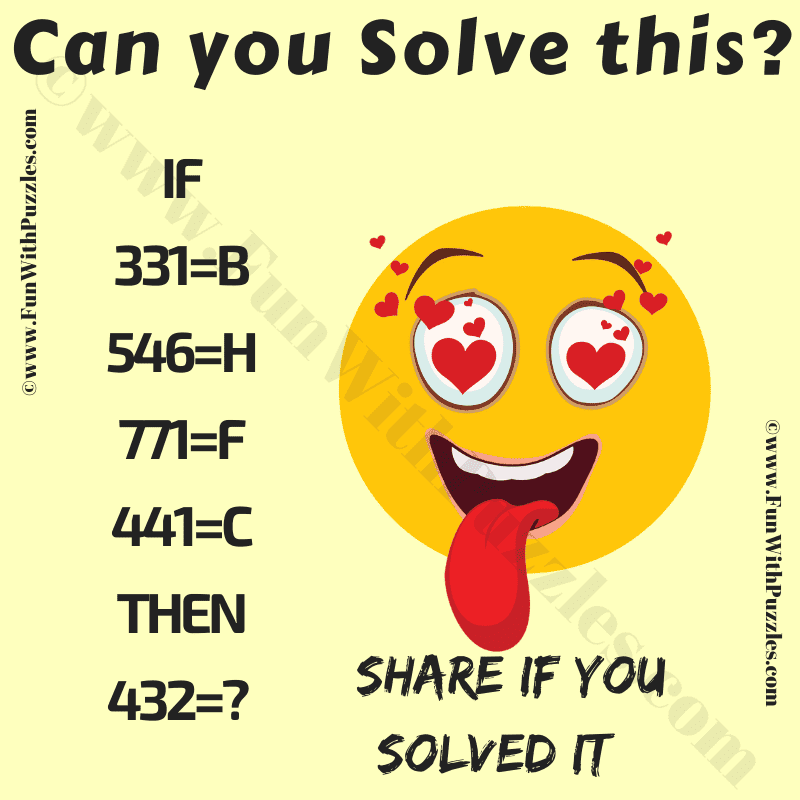This is a very interesting riddle with mathematics and logical reasoning. This maths logic riddle is for school students. Solving these kinds of logical reasoning riddles will help you to improve your logical reasoning skills. In this riddle, your challenge is to find the hidden logic in the given number equations. Once you are able to find the logic, try to find the missing number in the last equation. Write down your answer to the logical riddle in the comments.Can you solve this Maths Logic Riddle?

The answer to this "Riddle with Maths and Logic", can be viewed by clicking on the answer button.